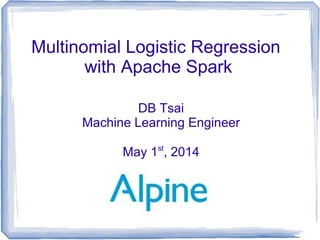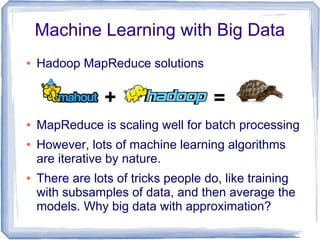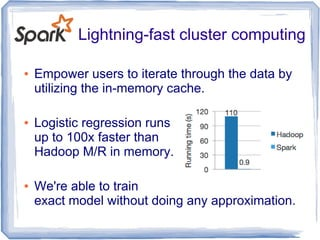Successfully reported this slideshow.

# Multinomial Logistic Regression with Apache Spark

22

Share×
1 of 31
1 of 31

# Multinomial Logistic Regression with Apache Spark

22

Share

Logistic Regression can not only be used for modeling binary outcomes but also multinomial outcome with some extension. In this talk, DB will talk about basic idea of binary logistic regression step by step, and then extend to multinomial one. He will show how easy it's with Spark to parallelize this iterative algorithm by utilizing the in-memory RDD cache to scale horizontally (the numbers of training data.) However, there is mathematical limitation on scaling vertically (the numbers of training features) while many recent applications from document classification and computational linguistics are of this type. He will talk about how to address this problem by L-BFGS optimizer instead of Newton optimizer.

Bio:
DB Tsai is a machine learning engineer working at Alpine Data Labs. He is recently working with Spark MLlib team to add support of L-BFGS optimizer and multinomial logistic regression in the upstream. He also led the Apache Spark development at Alpine Data Labs. Before joining Alpine Data labs, he was working on large-scale optimization of optical quantum circuits at Stanford as a PhD student.

Logistic Regression can not only be used for modeling binary outcomes but also multinomial outcome with some extension. In this talk, DB will talk about basic idea of binary logistic regression step by step, and then extend to multinomial one. He will show how easy it's with Spark to parallelize this iterative algorithm by utilizing the in-memory RDD cache to scale horizontally (the numbers of training data.) However, there is mathematical limitation on scaling vertically (the numbers of training features) while many recent applications from document classification and computational linguistics are of this type. He will talk about how to address this problem by L-BFGS optimizer instead of Newton optimizer.

Bio:
DB Tsai is a machine learning engineer working at Alpine Data Labs. He is recently working with Spark MLlib team to add support of L-BFGS optimizer and multinomial logistic regression in the upstream. He also led the Apache Spark development at Alpine Data Labs. Before joining Alpine Data labs, he was working on large-scale optimization of optical quantum circuits at Stanford as a PhD student.

## More Related Content

### Related Books

Free with a 14 day trial from Scribd

See all

### Related Audiobooks

Free with a 14 day trial from Scribd

See all

### Multinomial Logistic Regression with Apache Spark

1. 1. Multinomial Logistic Regression with Apache Spark DB Tsai Machine Learning Engineer May 1st , 2014
2. 2. Machine Learning with Big Data ● Hadoop MapReduce solutions ● MapReduce is scaling well for batch processing ● However, lots of machine learning algorithms are iterative by nature. ● There are lots of tricks people do, like training with subsamples of data, and then average the models. Why big data with approximation? + =
3. 3. Lightning-fast cluster computing ● Empower users to iterate through the data by utilizing the in-memory cache. ● Logistic regression runs up to 100x faster than Hadoop M/R in memory. ● We're able to train exact model without doing any approximation.
4. 4. Binary Logistic Regression d = ax1+bx2+cx0 √a2 +b2 where x0=1 P( y=1∣⃗x , ⃗w)= exp(d ) 1+exp(d ) = exp(⃗x ⃗w) 1+exp(⃗x ⃗w) P ( y=0∣⃗x , ⃗w)= 1 1+exp(⃗x ⃗w) log P ( y=1∣⃗x , ⃗w) P( y=0∣⃗x , ⃗w) =⃗x ⃗w w0= c √a2 +b2 w1= a √a2 +b2 w2= b √a2 +b2 wherew0 iscalled as intercept
5. 5. Training Binary Logistic Regression ● Maximum Likelihood estimation From a training data and labels ● We want to find that maximizes the likelihood of data defined by ● We can take log of the equation, and minimize it instead. The Log-Likelihood becomes the loss function. X =( ⃗x1 , ⃗x2 , ⃗x3 ,...) Y =( y1, y2, y3, ...) ⃗w L( ⃗w , ⃗x1, ... , ⃗xN )=P ( y1∣⃗x1 , ⃗w)P ( y2∣⃗x2 , ⃗w)... P( yN∣ ⃗xN , ⃗w) l( ⃗w ,⃗x)=log P(y1∣⃗x1 , ⃗w)+log P( y2∣⃗x2 , ⃗w)...+log P( yN∣ ⃗xN , ⃗w)
6. 6. Optimization ● First Order Minimizer Require loss, gradient of loss function – Gradient Decent is step size – Limited-memory BFGS (L-BFGS) – Orthant-Wise Limited-memory Quasi-Newton (OWLQN) – Coordinate Descent (CD) – Trust Region Newton Method (TRON) ● Second Order Minimizer Require loss, gradient and hessian of loss function – Newton-Raphson, quadratic convergence. Fast! ● Ref: Journal of Machine Learning Research 11 (2010) 3183- 3234, Chih-Jen Lin et al. ⃗wn+1=⃗wn−γ ⃗G , γ ⃗wn+1=⃗wn−H−1 ⃗G
7. 7. Problem of Second Order Minimizer ● Scale horizontally (the numbers of training data) by leveraging Spark to parallelize this iterative optimization process. ● Don't scale vertically (the numbers of training features). Dimension of Hessian is ● Recent applications from document classification and computational linguistics are of this type. dim(H )=[(k−1)(n+1)] 2 where k is numof class ,nis num of features
8. 8. L-BFGS ● It's a quasi-Newton method. ● Hessian matrix of second derivatives doesn't need to be evaluated directly. ● Hessian matrix is approximated using gradient evaluations. ● It converges a way faster than the default optimizer in Spark, Gradient Decent. ● We love open source! Alpine Data Labs contributed our L-BFGS to Spark, and it's already merged in Spark-1157.
9. 9. Training Binary Logistic Regression l( ⃗w ,⃗x) = ∑ k=1 N log P( yk∣⃗xk , ⃗w) = ∑k=1 N yk log P ( yk=1∣⃗xk , ⃗w)+(1−yk )log P ( yk =0∣⃗xk , ⃗w) = ∑k=1 N yk log exp( ⃗xk ⃗w) 1+exp( ⃗xk ⃗w) +(1−yk )log 1 1+exp( ⃗xk ⃗w) = ∑k=1 N yk ⃗xk ⃗w−log(1+exp( ⃗xk ⃗w)) Gradient : Gi (⃗w ,⃗x)= ∂l( ⃗w ,⃗x) ∂ wi =∑k=1 N yk xki− exp( ⃗xk ⃗w) 1+exp( ⃗xk ⃗w) xki Hessian: H ij (⃗w ,⃗x)= ∂∂l( ⃗w ,⃗x) ∂wi ∂w j =−∑k=1 N exp( ⃗xk ⃗w) (1+exp( ⃗xk ⃗w))2 xki xkj
10. 10. Overfitting P ( y=1∣⃗x , ⃗w)= exp(zd ) 1+exp(zd ) = exp(⃗x ⃗w) 1+exp(⃗x ⃗w)
11. 11. Regularization ● The loss function becomes ● The loss function of regularizer doesn't depend on data. Common regularizers are – L2 Regularization: – L1 Regularization: ● L1 norm is not differentiable at zero! ltotal (⃗w ,⃗x)=lmodel (⃗w ,⃗x)+lreg( ⃗w) lreg (⃗w)=λ∑i=1 N wi 2 lreg (⃗w)=λ∑i=1 N ∣wi∣ ⃗G (⃗w ,⃗x)total=⃗G(⃗w ,⃗x)model+⃗G( ⃗w)reg ̄H ( ⃗w ,⃗x)total= ̄H (⃗w ,⃗x)model+ ̄H (⃗w)reg
12. 12. Mini School of Spark APIs ● map(func) : Return a new distributed dataset formed by passing each element of the source through a function func. ● reduce(func) : Aggregate the elements of the dataset using a function func (which takes two arguments and returns one). The function should be commutative and associative so that it can be computed correctly in parallel. No “key” thing here compared with Hadoop M/R.
13. 13. Example – No More “Word Count”! Let's compute the mean of numbers. 3.0 2.0 1.0 5.0 Executor 1 Executor 2 map map (1.0, 1) (5.0, 1) (3.0, 1) (2.0, 1) reduce (5.0, 2) reduce (11.0, 4) Shuffle
14. 14. ● The previous example using map(func) will create new tuple object, which may cause Garbage Collection issue. ● Like the combiner in Hadoop Map Reduce, for each tuple emitted from map(func), there is no guarantee that they will be combined locally in the same executor by reduce(func). It may increase the traffic in shuffle phase. ● In Hadoop, we address this by in-mapper combiner or aggregating the result in global variables which have scope to entire partition. The same approach can be used in Spark.
15. 15. Mini School of Spark APIs ● mapPartitions(func) : Similar to map, but runs separately on each partition (block) of the RDD, so func must be of type Iterator[T] => Iterator[U] when running on an RDD of type T. ● This API allows us to have global variables on entire partition to aggregate the result locally and efficiently.
16. 16. Better Mean of Numbers Implementation 3.0 2.0 Executor 1 reduce (11.0, 4) Shuffle var counts = 0 var sum = 0.0 loop var counts = 2 var sum = 5.0 3.0 2.0 (5.0, 2) 1.0 5.0 Executor 2 var counts = 0 var sum = 0.0 loop var counts = 2 var sum = 6.0 1.0 5.0 (6.0, 2) mapPartitions
17. 17. More Idiomatic Scala Implementation ● aggregate(zeroValue: U)(seqOp: (U, T) => U, combOp: (U, U) => U): U zeroValue is a neutral "zero value" with type U for initialization. This is analogous to in previous example. var counts = 0 var sum = 0.0 seqOp is function taking (U, T) => U, where U is aggregator initialized as zeroValue. T is each line of values in RDD. This is analogous to mapPartition in previous example. combOp is function taking (U, U) => U. This is essentially combing the results between different executors. The functionality is the same as reduce(func) in previous example.
18. 18. Approach of Parallelization in Spark Spark Driver JVM Time Spark Executor 1 JVM Spark Executor 2 JVM 1) Find available resources in cluster, and launch executor JVMs. Also initialize the weights. 2) Ask executors to load the data into executors' JVMs 2) Trying to load the data into memory. If the data is bigger than memory, it will be partial cached. (The data locality from source will be taken care by Spark) 3) Ask executors to compute loss, and gradient of each training sample (each row) given the current weights. Get the aggregated results after the Reduce Phase in executors. 5) If the regularization is enabled, compute the loss and gradient of regularizer in driver since it doesn't depend on training data but only depends on weights. Add them into the results from executors. 3) Map Phase: Compute the loss and gradient of each row of training data locally given the weights obtained from the driver. Can either emit each result or sum them up in local aggregators. 4) Reduce Phase: Sum up the losses and gradients emitted from the Map Phase Taking a rest! 6) Plug the loss and gradient from model and regularizer into optimizer to get the new weights. If the differences of weights and losses are larger than criteria, GO BACK TO 3) Taking a rest! 7) Finish the model training! Taking a rest!
19. 19. Step 3) and 4) ● This is the implementation of step 3) and 4) in MLlib before Spark 1.0 ● gradient can have implementations of Logistic Regression, Linear Regression, SVM, or any customized cost function. ● Each training data will create new “grad” object after gradient.compute.
20. 20. Step 3) and 4) with mapPartitions
21. 21. Step 3) and 4) with aggregate ● This is the implementation of step 3) and 4) in MLlib in coming Spark 1.0 ● No unnecessary object creation! It's helpful when we're dealing with large features training data. GC will not kick in the executor JVMs.
22. 22. Extension to Multinomial Logistic Regression ● In Binary Logistic Regression ● For K classes multinomial problem where labels ranged from [0, K-1], we can generalize it via ● The model, weights becomes (K-1)(N+1) matrix, where N is number of features. log P( y=1∣⃗x , ̄w) P ( y=0∣⃗x , ̄w) =⃗x ⃗w1 log P ( y=2∣⃗x , ̄w) P ( y=0∣⃗x , ̄w) =⃗x ⃗w2 ... log P ( y=K −1∣⃗x , ̄w) P ( y=0∣⃗x , ̄w) =⃗x ⃗wK −1 log P( y=1∣⃗x , ⃗w) P ( y=0∣⃗x , ⃗w) =⃗x ⃗w ̄w=(⃗w1, ⃗w2, ... , ⃗wK −1)T P( y=0∣⃗x , ̄w)= 1 1+∑ i=1 K −1 exp(⃗x ⃗wi ) P( y=1∣⃗x , ̄w)= exp(⃗x ⃗w2) 1+∑ i=1 K −1 exp(⃗x ⃗wi) ... P( y=K −1∣⃗x , ̄w)= exp(⃗x ⃗wK −1) 1+∑i=1 K −1 exp(⃗x ⃗wi )
23. 23. Training Multinomial Logistic Regression l( ̄w ,⃗x) = ∑ k=1 N log P( yk∣⃗xk , ̄w) = ∑k=1 N α( yk)log P( y=0∣⃗xk , ̄w)+(1−α( yk ))log P ( yk∣⃗xk , ̄w) = ∑k=1 N α( yk)log 1 1+∑ i=1 K −1 exp(⃗x ⃗wi) +(1−α( yk ))log exp(⃗x ⃗w yk ) 1+∑ i=1 K−1 exp(⃗x ⃗wi) = ∑ k=1 N (1−α( yk ))⃗x ⃗wyk −log(1+∑ i=1 K−1 exp(⃗x ⃗wi)) Gradient : Gij ( ̄w ,⃗x)= ∂l( ̄w ,⃗x) ∂wij =∑k=1 N (1−α( yk )) xkj δi , yk − exp( ⃗xk ⃗w) 1+exp( ⃗xk ⃗w) xkj α( yk )=1 if yk=0 α( yk )=0 if yk≠0 Define: Note that the first index “i” is for classes, and the second index “j” is for features. Hessian: H ij ,lm( ̄w ,⃗x)= ∂∂l ( ̄w ,⃗x) ∂wij ∂wlm
24. 24. 0 5 10 15 20 25 30 35 0.3 0.35 0.4 0.45 0.5 0.55 0.6 0.65 0.7 Logistic Regression with a9a Dataset (11M rows, 123 features, 11% non-zero elements) 16 executors in INTEL Xeon E3-1230v3 32GB Memory * 5 nodes Hadoop 2.0.5 alpha cluster L-BFGS Dense Features L-BFGS Sparse Features GD Sparse Features GD Dense Features Seconds Log-Likelihood/NumberofSamples a9a Dataset Benchmark
25. 25. a9a Dataset Benchmark -1 1 3 5 7 9 11 13 15 0.3 0.35 0.4 0.45 0.5 0.55 0.6 0.65 0.7 Logistic Regression with a9a Dataset (11M rows, 123 features, 11% non-zero elements) 16 executors in INTEL Xeon E3-1230v3 32GB Memory * 5 nodes Hadoop 2.0.5 alpha cluster L-BFGS GD Iterations Log-Likelihood/NumberofSamples
26. 26. 0 5 10 15 20 25 30 0 0.1 0.2 0.3 0.4 0.5 0.6 0.7 0.8 Logistic Regression with rcv1 Dataset (6.8M rows, 677,399 features, 0.15% non-zero elements) 16 executors in INTEL Xeon E3-1230v3 32GB Memory * 5 nodes Hadoop 2.0.5 alpha cluster LBFGS Sparse Vector GD Sparse Vector Second Log-Likelihood/NumberofSamples rcv1 Dataset Benchmark
27. 27. news20 Dataset Benchmark 0 10 20 30 40 50 60 70 80 0 0.2 0.4 0.6 0.8 1 1.2 Logistic Regression with news20 Dataset (0.14M rows, 1,355,191 features, 0.034% non-zero elements) 16 executors in INTEL Xeon E3-1230v3 32GB Memory * 5 nodes Hadoop 2.0.5 alpha cluster LBFGS Sparse Vector GD Sparse Vector Second Log-Likelihood/NumberofSamples
28. 28. Alpine Demo
29. 29. Alpine Demo
30. 30. Alpine Demo
31. 31. Conclusion ● Alpine supports state of the art Cloudera CDH5 ● Spark runs programs 100x faster than Hadoop ● Spark turns iterative big data machine learning problems into single machine problems ● MLlib provides lots of state of art machine learning implementations, e.g. K-means, linear regression, logistic regression, ALS, collaborative filtering, and Naive Bayes, etc. ● Spark provides ease of use APIs in Java, Scala, or Python, and interactive Scala and Python shell ● Spark is Apache project, and it's the most active big data open source project nowadays.